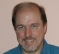## Proof of Z-transform of M-Fold Decimator/CompressorStarted by 6 years ago6 replieslatest reply 6 years ago184 views

Hello,

An M-Fold decimator is given by,

y(n) = x(nM)

Y(z) = Sum_-inf_to_inf [ x(nM) * z^-n ]

Pretty much every book that shows the derivation defines a comb sequence c(n) which is defined as follows:

c(n) = 1        , n = multiple of M

= 0        , otherwise

This new c(n) is basically a periodic delta(n) that repeats every M samples. I understand the entire derivation and how we get to the final result. However, the confusion for me is, why did this step have to occur?

What is the mathematical flaw in doing a "change of variable" in the Y(z) equation above, say, by defining p = nM? Of course by this approach we won't get to the same answer, so it has to be wrong. Moreover, defining c(n) makes sense if we are trying to keep it causal (now y(n) only depends on c(n)*x(n) instead of a future sample), but while deriving the math, should we worry about introducing causality artificially?

Why could Y(z) not be solved without defining this comb (and subsequently representing it by fourier series)?

Since decimation is basically the sample operation as sampling an analog signal, except that we now just sample a sampled signal,  decimation should also create shifted copies of the baseband frequency response. This is clearly shown by the approach in the textbook. So, I understand the result and it makes sense, whichever way it is derived. But the critical point in arriving at the result is the definition of the comb sequence c(n). So I want to understand how to think more intuitively about this step in the derivation.

-Srikar

[ - ]Intuition and math takes a lot of practice.  My favorite story is about a professor when asked if something was "obvious" - he walked out of the lectured with his hands on his chin and came back 15 minutes later and said "yes, it is obvious!"

One way to think about the comb is to expand the delta functions into exponential peaks.  As you squeeze the sides in, the peaks get higher, until at zero width they have infinite hight.  Whether you do sums or integrals doesn't really matter, the idea is that you are leaving more stuff out as you squeeze.

So if you start with all the samples, and then slowly reduce them in between every Mth sample, you will build up a picture of the comb times the original sample.  This is the path from everything to 1/M things - only instead of going slowly the text book just multiplies the source by the comb.

Asking questions is the best way to gain practice!

Dr. mike

[ - ]"Intuition and math takes a lot of practice."

I prefer the word "pruning".  When you start to approach these problems, some of your brain cells understand what's going on, and some simply refuse to be wrapped around it.  If you think about the problem hard enough and long enough, then those objecting brain cells will die from exasperation and be pruned.  The rest will understand, and find the answer obvious.

[ - ]Hey Srikar:

If you define p = nM and then try to do the transforms around z^(-p), then the index isn't going 0, 1, 2, etc.  Rather, it'll be going 0, M, 2M, etc.

I think you can go ahead and do this without the intermediate step of the sampler -- you should end up with something that's mathematically correct, but without the insight into what's happening to the frequency spectrum that the use of c(n) will provide.

[ - ]Hi Tim,

Thanks, I get it now. Yes, I like the approaches given in the books because they provide a very good intuition on the spectral behavior.

Regards,

Srikar

[ - ]